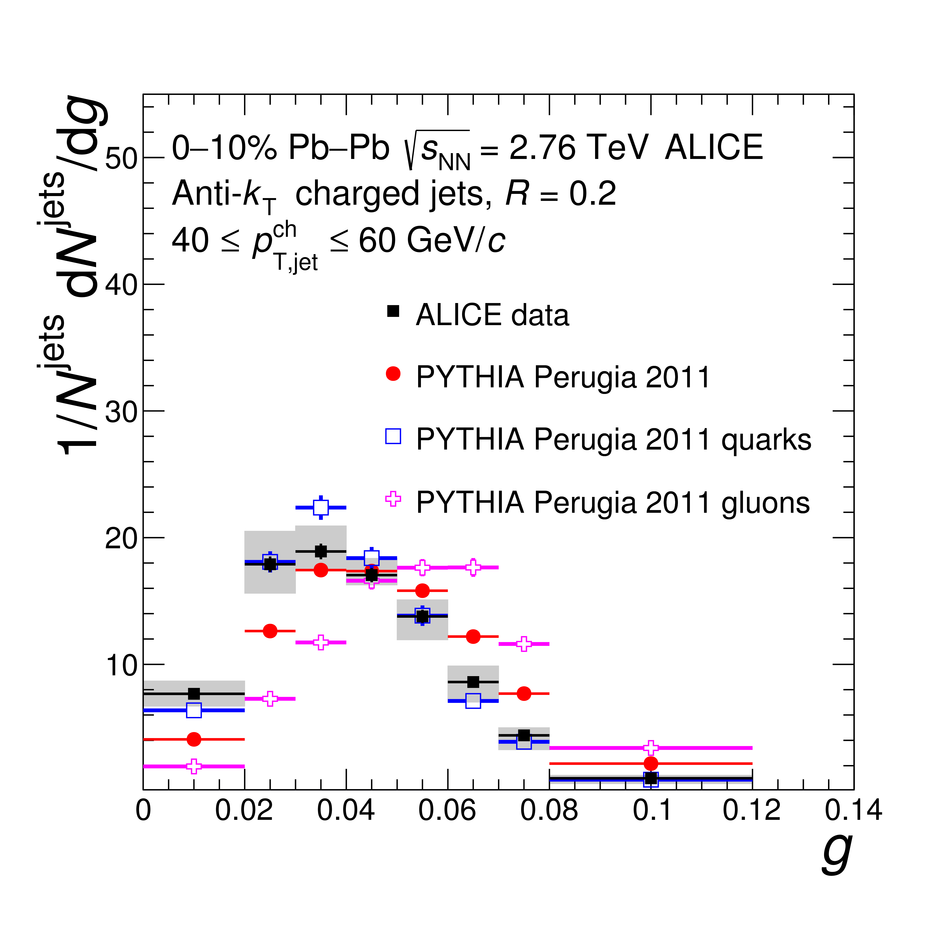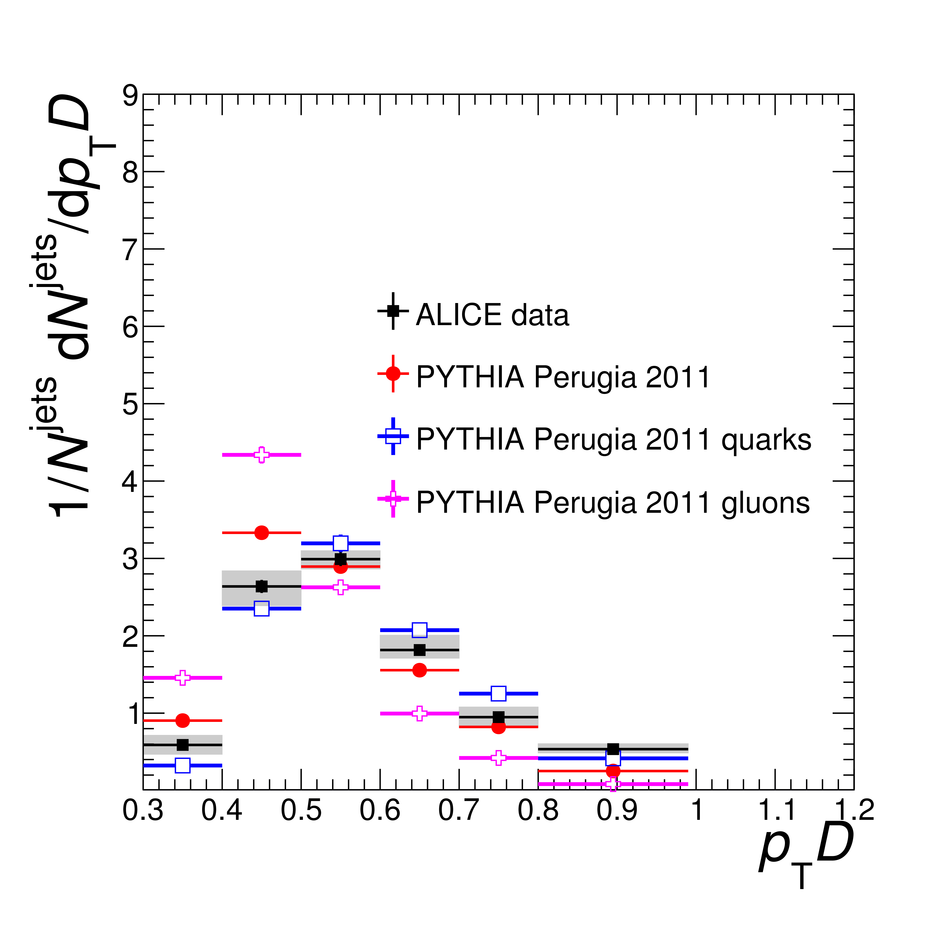# Figure 7

 Jet shape distributions in $0$--$10\%$ central Pb--Pb collisions at $\sqrt{s_{\rm NN}}=2.76$\,TeV for $R = 0.2$ in range of jet $p_{\mathrm{T,jet}}^{\rm ch}$ of $40$--$60$\,GeV$/c$ compared to quark and gluon vacuum generated jet shape distributions. The coloured boxes represent the experimental uncertainty on the jet shapes.